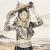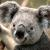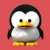php实现字符串反转

php实现字符串反转，不用strrev,不借用数组方式，时间复杂度度小于O(n)的，首尾交换的那种实现。

0<?php
\$a = "abcdefgh";
\$len = strlen(\$a);

\$revCnt = floor(\$len/2);
for (\$i = 0; \$i < \$revCnt; \$i++) {
\$temp = \$a{\$i};
\$a{\$i} =  \$a{\$len-1-\$i};
\$a{\$len-1-\$i} = \$temp;
}

echo \$a;

1PHP提供那么丰富的数组和字符串处理函数，就是方便编程者使用的，如果想在PHP上耍算法，出门右拐找C去，因为PHP那些函数都是用C实现的。